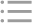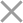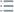To get a trial key
fill out the form below
** By clicking this button you agree to our Privacy Policy statementRequest our prices
--Select currency--
USD
EUR
* By clicking this button you agree to our Privacy Policy statementFree PVS-Studio license for Microsoft MVP specialists
** By clicking this button you agree to our Privacy Policy statementTo get the licence for your open-source project, please fill out this form
** By clicking this button you agree to our Privacy Policy statementI am interested to try it on the platforms:
 Windows Linux macOS PVS-Studio for .NET Core JetBrains Rider
** By clicking this button you agree to our Privacy Policy statementMessage submitted.

Your message has been sent. We will email you at

check your Spam/Junk folder and click the "Not Spam" button for our message.
This way, you won't miss messages from our team in the future.

>
>
>
V555. Expression of the 'A - B > 0' …Introduction
Analyzing projects
On Windows
On Linux and macOS
Continuous use of the analyzer in software development
Deploying the analyzer in cloud Continuous Integration services
Managing analysis results
Analyzer diagnostics
General Analysis (C++)
General Analysis (C#)
General Analysis (Java)
Diagnosis of micro-optimizations (C++)
Diagnosis of 64-bit errors (Viva64, C++)
Customer specific requests (C++)
MISRA errors
AUTOSAR errors
OWASP errors (C++)
OWASP errors (C#)
OWASP errors (Java)
Problems related to code analyzerContents

# V555. Expression of the 'A - B > 0' kind will work as 'A != B'.

Dec 20 2010

The analyzer detected a potential error in an expression of "A - B > 0" type. It is highly probable that the condition is wrong if the "A - B" subexpression has the unsigned type.

The "A - B > 0" condition holds in all the cases when 'A' is not equal to 'B'. It means that we may write the "A != B" expression instead of "A - B > 0". However, the programmer must have intended to implement quite a different thing.

Consider this sample:

``````unsigned int *B;
...
if (B[i]-70 > 0)``````

The programmer wanted to check whether the i-item of the B array is above 70. He could write it this way: "B[i] > 70". But he, proceeding from some reasons, wrote it this way: "B[i]-70 > 0" and made a mistake. He forgot that items of the 'B' array have the 'unsigned' type. It means that the "B[i]-70" expression has the 'unsigned' type too. So it turns out that the condition is always true except for the case when the 'B[i]' item equals to 70.

Let's clarify this case.

If 'B[i]' is above 70, then "B[i]-70" is above 0.

If 'B[i]' is below 70, then we will get an overflow of the unsigned type and a very large value as a result. Let B[i] == 50. Then "B[i]-70" = 50u - 70u = 0xFFFFFFECu = 4294967276. Surely, 4294967276 > 0.

A demonstration sample:

``````unsigned A;
A = 10; cout << "A=10 " << (A-70 > 0) << endl;
A = 70; cout << "A=70 " << (A-70 > 0) << endl;
A = 90; cout << "A=90 " << (A-70 > 0) << endl;
// Will be printed
A=10 1
A=70 0
A=90 1``````

The first way to correct the code:

``````unsigned int *B;
...
if (B[i] > 70)``````

The second way to correct the code:

``````int *B;
...
if (B[i]-70 > 0)``````

Note that an expression of the "A - B > 0" type far not always signals an error. Consider a sample where the analyzer generates a false alarm:

``````// Functions GetLength() and GetPosition() return
// value of size_t type.
while ((inStream.GetLength() - inStream.GetPosition()) > 0)
{ ... }``````

GetLength() is always above or equal to GetPosition() here, so the code is correct. To suppress the false alarm, we may add the comment //-V555 or rewrite the code in the following way:

``````while (inStream.GetLength() != inStream.GetPosition())
{ ... }``````

Here is another case when no error occurs.

``````__int64 A;
__uint32 B;
...
if (A - B > 0)``````

The "A - B" subexpression here has the signed type __int64 and no error occurs. The analyzer does not generate warnings in such cases.

This diagnostic is classified as:

 CWE-191CERT-INT02-C
 You can look at examples of errors detected by the V555 diagnostic.